Math & Scientific Tools software reviews

Showing 26 to 50 from 132 matches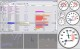OneCable.net - Explorer 2.3.12.9 Math & Scientific Tools SMS-X10-PC-remote-control logger for sensors, actors and GPS with mobile phones Shareware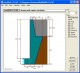LeanWall 1.10.40 Math & Scientific Tools A software for the purpose of designing a concrete or masonry leaning wall. Demo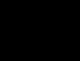Numap7 7.06 Math & Scientific Tools Freeware for fast development and application of approximation type networks FreewareNuclass7 7.06 Math & Scientific Tools Freeware for fast development,application of neural and conventional classifiers Freeware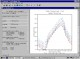ComfortAir HVAC Software 4.0 Math & Scientific Tools Heating and air conditioning hourly load calculation and analysis. Demo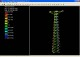MATruss 1.5 Math & Scientific Tools MATruss performs strength calculations for truss constructions. SharewareEngCalc(Automotive)- PocketPC Calculator 2.0 Math & Scientific Tools EngCalc (Automotive) - Automobile Engineers Engineering Formula Calculator DemoVIMeteo 1.2 Math & Scientific Tools Real-time weather data from 7000 stations all around the world. Demo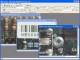IC Capture - image capture application 2.0 Math & Scientific Tools Windows application to capture images from WDM stream class devices. Commercial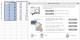xlDEA 2.1 Math & Scientific Tools Perform Data Envelopment Analysis in MS Excel - with customizable Flash charts. SharewareThermalWall 2.0.0.1 Math & Scientific Tools Calculus of fundamental dynamic thermal characteristics of a multilayer wall. Freeware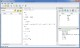Doronix Math Toolbox 2.0 Math & Scientific Tools Doronix Math Toolbox is a numerical computing environment similar to MATLAB SharewareVIGraph 1.5 Math & Scientific Tools Graph builder for your txt data files Demo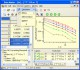Data Master 2003 11.8.0.433 Math & Scientific Tools Plot, edit and analyze numeric data, automate measurements and post-process FreewareCASC concentration calculator 1.0.2.35 Math & Scientific Tools CASC calculates recipes for solution preparation and converts concentrations Shareware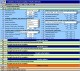MITCalc - Shaft connection 1.21 Math & Scientific Tools Designs and strength checks of shaped connections of shafts with hubs Shareware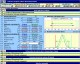MITCalc - Tolerance analysis 1.17 Math & Scientific Tools Tolerance analysis of linear, 2D and 3D dimensional chains Shareware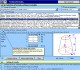MITCalc - Technical Formulas 1.19 Math & Scientific Tools Technical formulas (Solids, motion, friction...) / Units convertor Shareware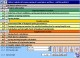MITCalc - Tension Springs 1.20 Math & Scientific Tools Geometric and strength designs of helical tension cylindrical springs Shareware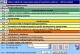MITCalc - Compression Springs 1.20 Math & Scientific Tools Geometric and strength designs of helical compression cylindrical springs Shareware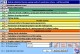MITCalc - Torsion Springs 1.20 Math & Scientific Tools Geometric and strength designs of spiral cylindrical torsion springs Shareware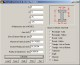Engineering Power Tools 1.9.8 Math & Scientific Tools Engineering software solves common engineering problems quickly & efficiently. Shareware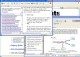WebCab Probability and Stat (J2EE Ed.) 3.6 Math & Scientific Tools Statistics, Discrete Prob, Distributions, Hypo. testing, Correlation,Regression Demo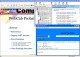WebCab Probability and Stat for .NET 3.6 Math & Scientific Tools Statistics, Discrete Prob, Distributions, Hypo. testing, Correlation,Regression Demo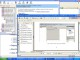WebCab Probability and Stat for Delphi 3.6 Math & Scientific Tools Statistics, Discrete Prob, Distributions, Hypo. testing, Correlation,Regression Demo